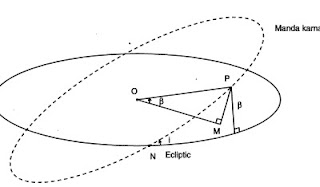The Kerala School of Astronomy and Maths   By Govind Kumar

The Indian astronomers were interested in the computation of eclipses, of geocentric longitudes, the risings and settings of planets,which had relevance to the day to day activities of people. The Kerala School of Astronomy and Mathematics ( KSAM ) was founded by Madhava of Sangramagrama and it was followed by brilliant mathematicians, Parameswara, Jyeshta Deva, Neelakanta, Achyuta Pisharadi, Neelakanta, Sankara Varier et al.

Did not Emerson say?

"Astronomy is excellent, it should come down and give life its full value, and not rest amidst globes and spheres ".

They were not bothered about proposing Models of the Universe and gaining publicity. But then they did discuss the geometrical model, the rationale of their computations.The above diagram explains the Geometric Model of Parameswara, another Kerala astronomer. Paramesvara and Nilakanta modified the Aryabhatan Model.

By Sheegroccha, he meant the longitude of the Sun." Sheegrocham Sarvesham Ravir bhavathi ", he says is his book Bhatadeepika . For the interior planets, the longitude of the Sheegra correction is to be deducted from the Sun's longitude, Ravi Sphuta to get the Anomaly of Conjunction.

The Manda Prathimandala is the mean angular motion of the Planet, from which the trignometric corrections are given to get the true, geocentric longitude.

New Page 1
Jyeshtadeva was a Kerala astronomer who helped in the calculation of longitudes, when there is latitudinal deflection. In his Yukti Bhasa, he calculates correctly the cos l, the cosine of latitude, which is important in the Reduction to the Ecliptic.

There is a separate section in the Yukti Bhasa, which deals with the effects of the inclination of a planet's orbit on its latitude. He describes how to find the true longitude of a planet, Sheegra Sphutam, when there is latitudinal deflection.

"Now calculate the Vikshepa Koti, cos l, by subtracting the square of the Vikshepa from the square of the Manda Karna Vyasardha and calculating the root of the difference."In the above diagram,

N is the Ascending Node
P is the planet on the Manda Karna Vritta, inclined to the Ecliptic

Vikshepa Koti = OM = ( OP^2 - PM^2 ) ^1/2

Taking this Vikshepa Koti and assuming it to be the Manda Karna, sheegra sphuta, the true longitude, has to be calculated as before.

Astronomy and Maths are related to Astrology. Emerson opined that " Astrology is Astronomy brought down to Earth, into the affairs of men" !